Search by Topic

Filter by: Content type:
Age range:
Challenge level:

There are 28 results

Broad Topics > Numbers and the Number System > Number bases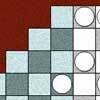Napier's Location Arithmetic

Age 14 to 16 Challenge Level:

Have you seen this way of doing multiplication ?Guesswork

Age 14 to 16 Challenge Level:

Ask a friend to choose a number between 1 and 63. By identifying which of the six cards contains the number they are thinking of it is easy to tell them what the number is.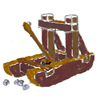Oh for the Mathematics of Yesteryear

Age 11 to 14 Challenge Level:

A garrison of 600 men has just enough bread ... but, with the news that the enemy was planning an attack... How many ounces of bread a day must each man in the garrison be allowed, to hold out 45. . . .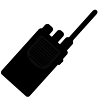Secret Transmissions

Age 14 to 16 Challenge Level:

How can Agent X transmit data on a faulty line and be sure that her message will get through?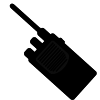More Secret Transmissions

Age 16 to 18 Challenge Level:

In 'Secret Transmissions', Agent X could send four-digit codes error free. Can you devise an error-correcting system for codes with more than four digits?Fingers and Hands

Age 7 to 11 Challenge Level:

How would you count the number of fingers in these pictures?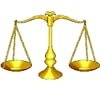Balance Power

Age 11 to 18 Challenge Level:

Using balancing scales what is the least number of weights needed to weigh all integer masses from 1 to 1000? Placing some of the weights in the same pan as the object how many are needed?Alien Counting

Age 7 to 11 Challenge Level:

Investigate the different ways these aliens count in this challenge. You could start by thinking about how each of them would write our number 7.Age 7 to 18

This article for the young and old talks about the origins of our number system and the important role zero has to play in it.The Knapsack Problem and Public Key Cryptography

Age 16 to 18

An example of a simple Public Key code, called the Knapsack Code is described in this article, alongside some information on its origins. A knowledge of modular arithmetic is useful.Knapsack

Age 14 to 16 Challenge Level:

You have worked out a secret code with a friend. Every letter in the alphabet can be represented by a binary value.Composite Notions

Age 14 to 16 Challenge Level:

A composite number is one that is neither prime nor 1. Show that 10201 is composite in any base.Phew I'm Factored

Age 14 to 16 Challenge Level:

Explore the factors of the numbers which are written as 10101 in different number bases. Prove that the numbers 10201, 11011 and 10101 are composite in any base.Age 11 to 18

We are used to writing numbers in base ten, using 0, 1, 2, 3, 4, 5, 6, 7, 8, and 9. Eg. 75 means 7 tens and five units. This article explains how numbers can be written in any number base.Nim-interactive

Age 11 to 16 Challenge Level:

Start with any number of counters in any number of piles. 2 players take it in turns to remove any number of counters from a single pile. The winner is the player to take the last counter.Nim-like Games

Age 7 to 16 Challenge Level:

A collection of games on the NIM theme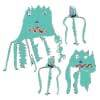Back to the Planet of Vuvv

Age 11 to 14 Challenge Level:

There are two forms of counting on Vuvv - Zios count in base 3 and Zepts count in base 7. One day four of these creatures, two Zios and two Zepts, sat on the summit of a hill to count the legs of. . . .Back to Basics

Age 14 to 16 Challenge Level:

Find b where 3723(base 10) = 123(base b).Basically

Age 11 to 14 Challenge Level:

The number 3723(in base 10) is written as 123 in another base. What is that base?Happy Octopus

Age 11 to 14 Challenge Level:

This investigation is about happy numbers in the World of the Octopus where all numbers are written in base 8 ... Find all the fixed points and cycles for the happy number sequences in base 8.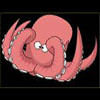Base Puzzle

Age 11 to 14 Challenge Level:

This investigation is about happy numbers in the World of the Octopus where all numbers are written in base 8 .Octi the octopus counts.Latin Numbers

Age 14 to 16 Challenge Level:

Can you create a Latin Square from multiples of a six digit number?Basic Rhythms

Age 16 to 18 Challenge Level:

Explore a number pattern which has the same symmetries in different bases.Binary Squares

Age 16 to 18 Challenge Level:

If a number N is expressed in binary by using only 'ones,' what can you say about its square (in binary)?Nim

Age 14 to 16 Challenge Level:

Start with any number of counters in any number of piles. 2 players take it in turns to remove any number of counters from a single pile. The loser is the player who takes the last counter.Code to Zero

Age 16 to 18 Challenge Level:

Find all 3 digit numbers such that by adding the first digit, the square of the second and the cube of the third you get the original number, for example 1 + 3^2 + 5^3 = 135.It Must Be 2000

Age 7 to 11 Challenge Level:

Here are many ideas for you to investigate - all linked with the number 2000.Sometimes We Lose Things

Age 7 to 11 Challenge Level:

Well now, what would happen if we lost all the nines in our number system? Have a go at writing the numbers out in this way and have a look at the multiplications table.